Equations of Straight Lines MathBitsNotebook.com Terms of Use   Contact Person: Donna RobertsThings you need to know to work with equations of lines:
Slope formula based on coordinates:
(Slope is represented by "m")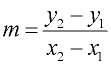If you need to refresh your knowledge of "slope", see Slope and Rate of Change.
Points on Lines:
If a point lies on a line, the coordinates of the point make the equation true.
Does (4,7) lie on the line y = 3x - 5?
Yes.
7 = 3(4) - 5
7 = 12 - 5
7 = 7 True.

 Different Ways to Write Equations of LinesSlope-Intercept Form:
 Slope-Intercept Form:   y = mx + b   (m = slope; b = y-intercept) • Use when you know the slope and the y-intercept (where the line crosses the y-axis), or are asked to find the slope and/or y-intercept. • Be sure your equation starts with "y =" to use this form. You will need to rewrite the equation if it does not start in this manner.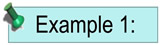Find the slope and y-intercept of the equation 4x + 2y = 6. Solution: Rewrite the equation to be "y =": y = -2x + 3 Match your new equation to the form y = mx + b Slope (m) is -2 and y-intercept (b) is +3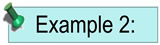Find the equation of a line whose slope is 5 and y-intercept is (0,-6). Solution: The given information tells us that m = 5 and b = -6 Place these values in the form y = mx + b Equation: y = 5x + (-6) or y = 5x - 6Point Slope Form:
 Point Slope Form:   y - y1= m (x - x1) m = slope; b = y-intercept;  (x1,y1) = any point on the line
• Use when you know a point on the line and the slope (or can determine the slope).

• Be sure to subtract the coordinates of your point on the line from y and from x.Find the equation of a line with a slope of -1 passing through (-4,6).

Solution: The given information tells us that m = -1 and (x1,y1) = (-4,6)
Substitute into the form y - y1 = m(x - x1):      y - 6 = -1(x - (-4))
Simplify:   y - 6 = -1(x + 4)   or   y - 6 = -x - 4   or   y = -x + 2   or   x + y = 2
(Your problem may specify what the equation should look like when you simplify.)Find the equation of a line passing through (4,-8) and (-2,6).
Solution: Find the slope first: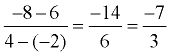Use either point: we will use (4,-8)
Substitute into the form y - y1 = m(x - x1):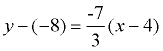Simplify: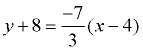or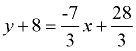or   3y = -7x + 4   or   7x + 3y = 4Standard Form:
 Standard Form:   Ax + By = C A and B cannot both be zero. Different textbooks have different interpretations of the name "standard form", and different statements of the form. You may see Standard Form called General Form. You may see the form written as Ax + By - C = 0.Find the Standard Form equation of a line through (-1,2) and (4,3).

Solution: Find the slope first: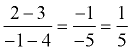Use either point: we will use (4,3)
Substitute into the form y - y1 = m(x - x1):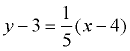Change to Standard Form:  x - 5y = -11Find the Standard Form of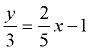.
Solution: Multiply All terms by the common denominator, 15.   5y = 6x - 15
Change to Standard Form:    6x - 5y = 15Horizontal Lines:
 Horizontal Line Form:   y = 7 (or any number) • Horizontal lines have a slope of zero because they have lots of "run", but no "rise". The rise/run formula always has a numerator of zero, making all of the ratios zero. • The actual formula is y = 0x + 7, but the 0x is not written. • This equation describes what is happening to all of the y-coordinates (all y-coordinates are 7).Find the equation of a line passing through the points (1,4) and (6,4). Solution: There are several ways to attack this problem. If you can picture that plotting these two points will create a horizontal line, your work is easy. Equation: y = 4Find the slope of a horizontal line passing through (-4,-8). Solution: All horizontal lines have a slope of zero. The point (-4,-8) is not needed (it is a distractor). Slope = 0.Vertical Lines:
 Vertical Line Form:   x = -3 (or any number) • Vertical lines have no slope (undefined) because they have lots of "rise", but no "run". The rise/run formula always has a denominator of zero, making all of the ratios undefined. • Vertical line formulas never contain a y-value. • This equation describes what is happening to all of the x-coordinates (all x-coordinates are -3).Find the equation of a line passing through the points (7,4) and (7,-2). Solution: There are several ways to attack this problem. If you can picture that plotting these two points will create a vertical line, your work is easy. Equation: x = 7Find the slope of a vertical line passing through (1,2). Solution: All vertical lines have no slope (or undefined slope). The slope does not exist. The point (1,2) is not needed (it is a distractor). Slope = undefined (does not exist).Using the Graphing Calculator:
While the graphing calculator can be helpful in working with any of the methods shown above, the calculator can also create the equation of a line given two points on the line. The calculator uses a statistics feature called a "Linear Regression" to give you the slope and y-intercept of y = ax + b (the calculator's version of y = mx + b). Follow the link below to see how this works.For calculator help with Creating Equations click here.